This project MLSE Application.osd demonstrates the application of the component ‘MLSE Equalizer’. The MLSE Equalizer component is available in the OptiSystem component library folder ‘Default/Receivers Library/Regenerators’ (Figure 1).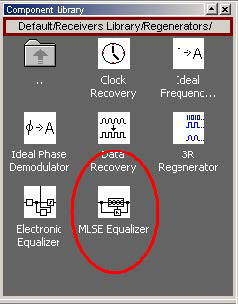Figure 1: OptiSystem component library

The system layout is presented in Figure 2. A 10 GB/s BPSK signal is generated by the ‘BPSK Generator’ component. The signal is then applied to a propagation channel. The propagation channel is an FIR filter with coefficients [1 0.8 0.3], that simulates a time dispersive channel that causes intersymbol interference (ISI). The signal is then applied to the MLSE equalizer component that will compensate the signal dispersion.
The channel estimation is done in the MLSE equalizer using the same coefficients as the FIR filter. The coefficients are loaded from a text file (FIR.dat -Figure 3).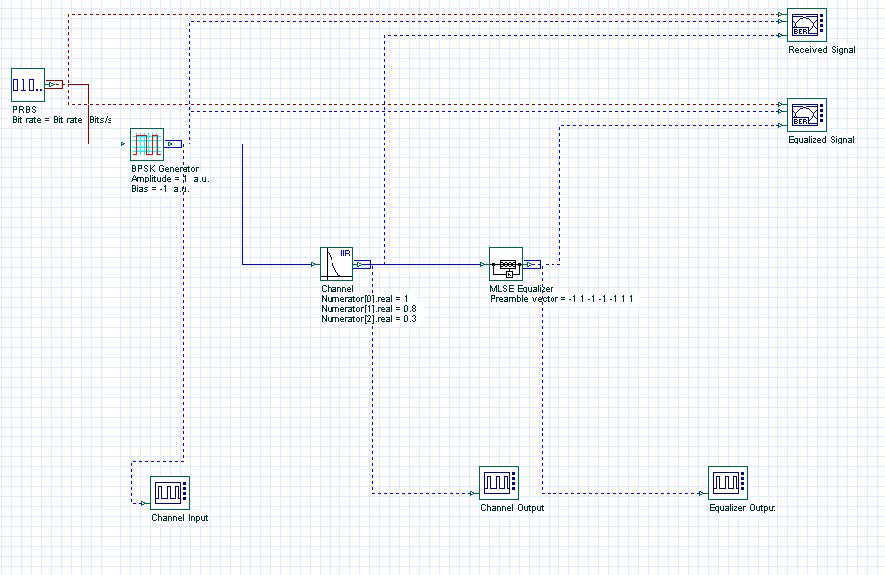Figure 2: System layout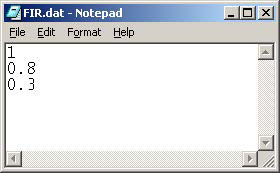Figure 3: Filter coefficients

The MLSE equalizer can also use an optional preamble. Figure 4 presents the eye diagram before and after the equalizer. Figure 5 presents the signal evolution from the channel input to the equalizer output. The equalizer mitigates ISI and improves the system’s performance.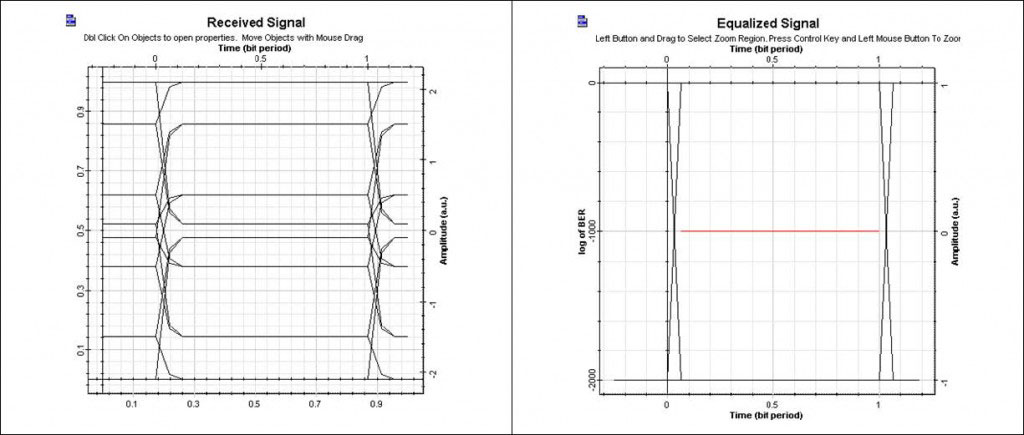Figure 4: Eye diagram before and after the equalizer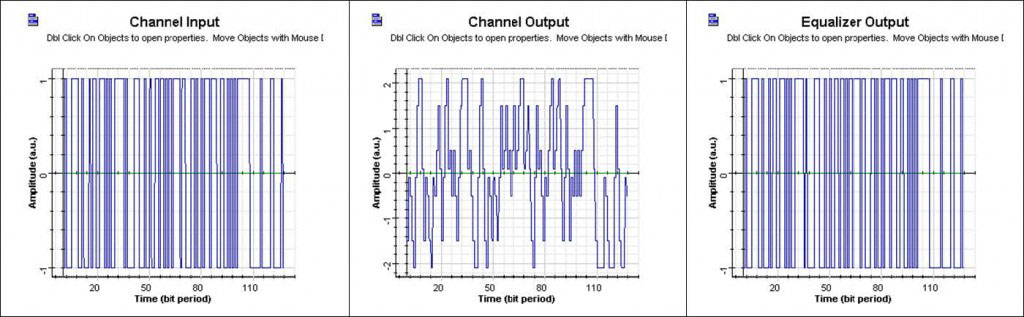Figure 5: Signal propagation in the system